# Debug Using Clojure Repl.

jaeyeon-jo-kr

26 Jan 2022• Clojure

One of biggest advantage of Clojure is getting instantly feedback using repl. Many Clojure IDE provides evaluation of code in editing in files. While writing In the source code, I wrote list and getting result instantly. Here's some tip about using repl.

## Basic

Let's start from printing "Hello world!". Writing this end press evaluation key(Ctrl + c, v, v In emacs)

``````(println "Hello World!")
``````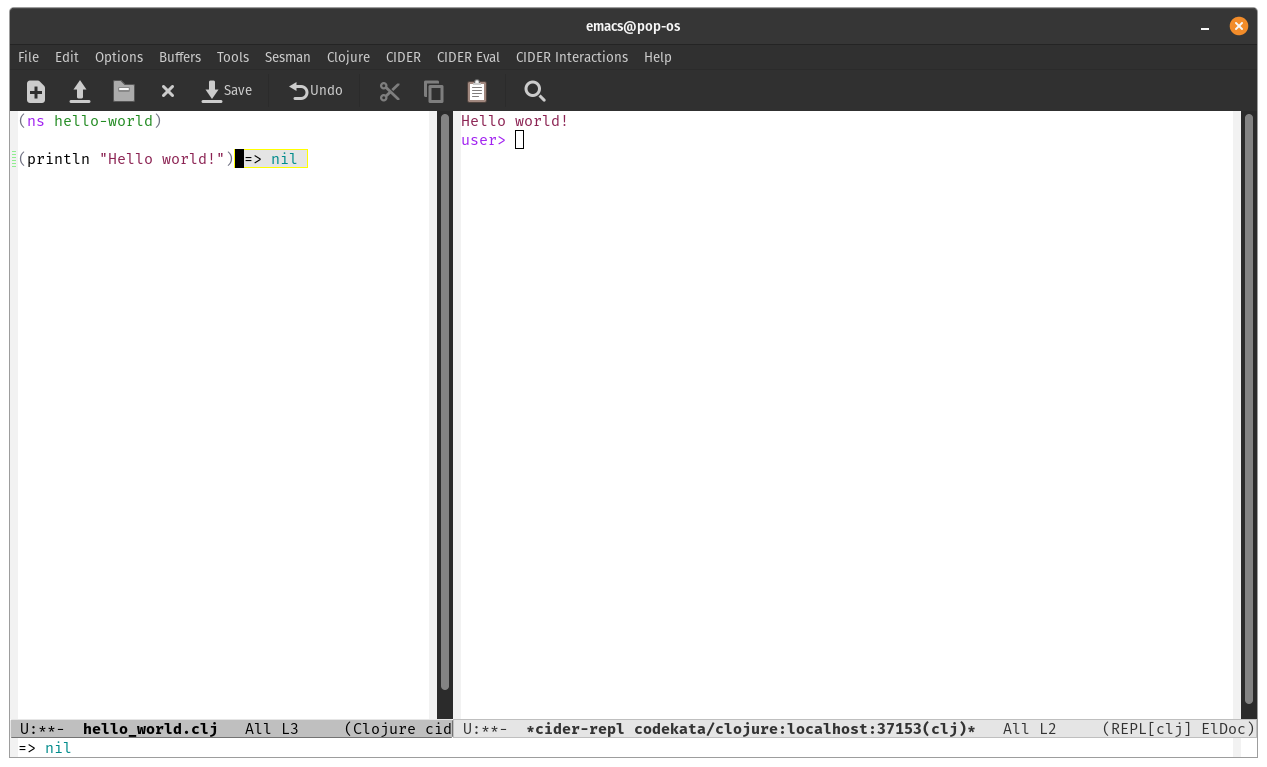The result returns nil, output is printed on repl browser. I want to view result on file, so I change the approach. Let's evaluate just string, and create clojure file and write "Hello World!" inside file.

``````"Hello World!"
``````

The results are below :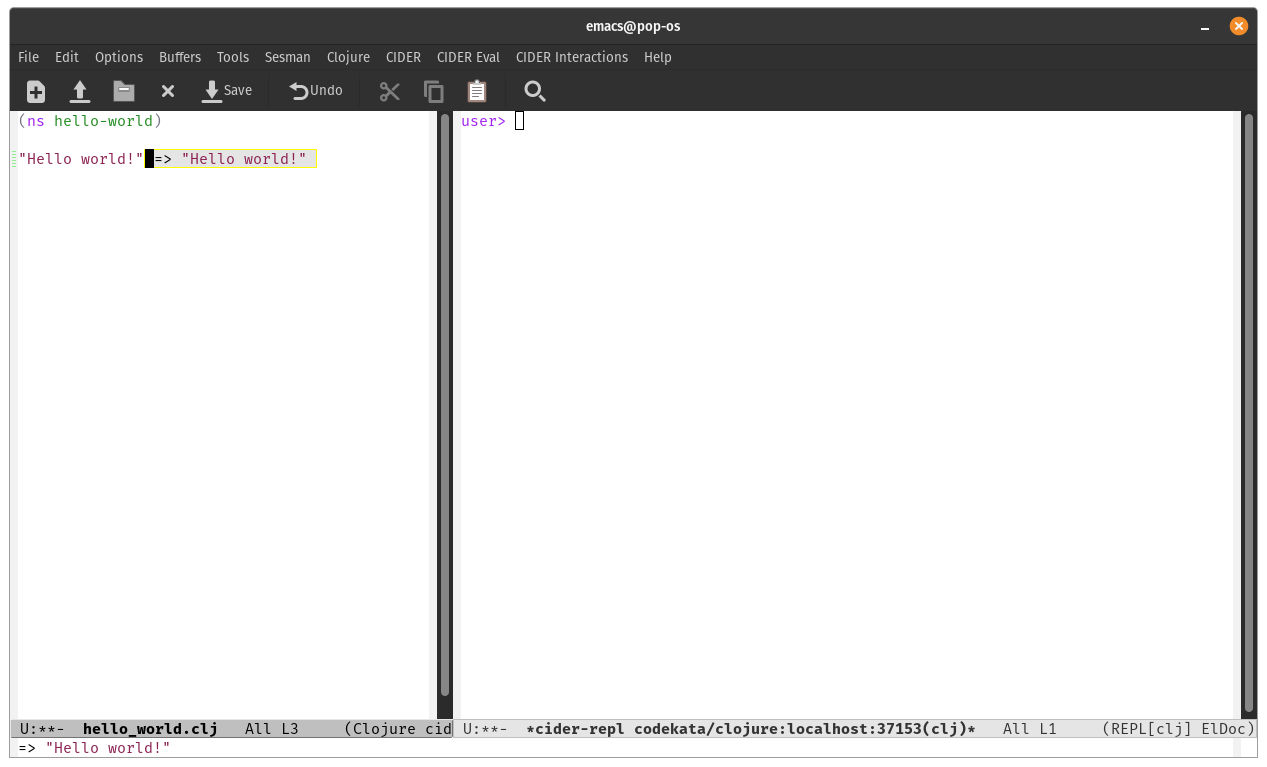The result is not showing on repl, but we can view on source file.

## Writing function to unit test

While writing a Clojure files in projects, I create many functions and changes. I want to view the result of changes function instantly. First, define function and evaluates.

``````(defn sum
[a b]
(+ a b))
``````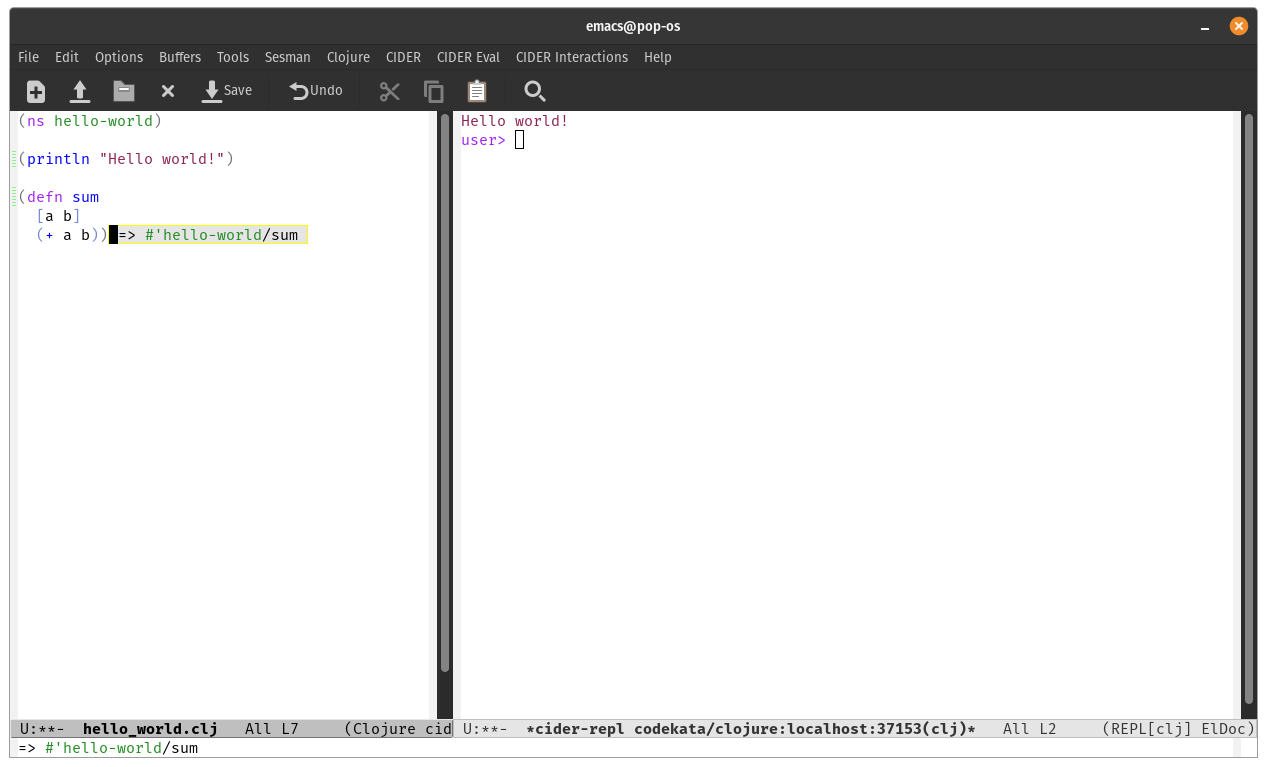The function is defined in clojure repl. To testing this function, just write evaluation expression below of function,. But writing test code in the comment block is recommended because the test cannot be executed while loading source files using loading namespaces.

I wrote like this:

``````(comment
(sum 1 2)
)
``````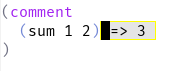When testing is finished, moving the test code to unit test. I think it's better than TDD.

``````(ns my-test.my-test
(:require [clojure.test :refer :all]))

(deftest sum-test
(testing "Testing sum"
(is (= 3 (sum 1 2)))))
``````

## Debugging chaining function

Calling multiple function is inevitable. I prefer to using -> or ->> thread in the chaining functions (Transducer and reducer is not familiar with me). Let's make simple chaining function.

``````(->> (range 1 10)
(map inc)
(filter odd?))
``````

OK, I can evaluate first row and last result of expression.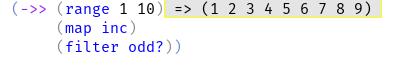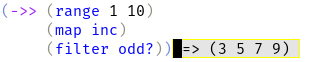But how to see the result of middle function? Comment the function using semi-colon.

``````(->> (range 1 10)
(map inc)
;(filter odd?)
)
``````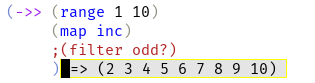That's it! Using ->, ->> or combining function, we can show results fast without debugger.

## Testing lambda in function

It' ideally perfect if all of functions are tested independently. But in case of lambda, it sometimes depends on nesting functions. Let's see this function :

``````(defn sum-with-multiple
[a b c]
(let [f (fn [d] (* d c))]
(f (+ a b))))

``````

How to test 'f'? Print the result of function.

``````(defn sum-with-multiple
[a b c]
(let [f (fn [d] (* d c))]
(println (f (+ a b)))
(f (+ a b))))

``````

The function is calling twice. If the function has side effect, the result will be changed. Let's using binding.

``````(defn sum-with-multiple
[a b c]
(let [f (fn [d] (* d c))
result (f (+ a b))]
(println result)
result))
``````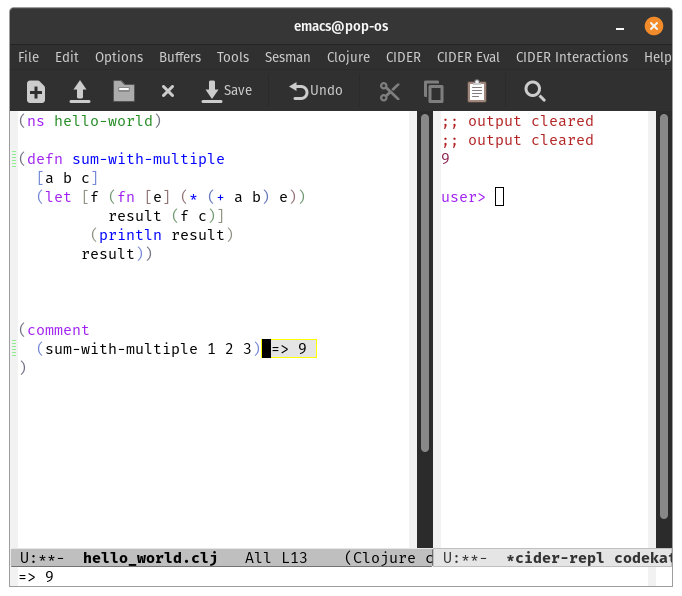I see print result and show final result. But changes many code. And if another function is added and executed, the situation is worse.

Let's try evaluate lambda function which is part of sum-with-multiple.

``````(defn sum-with-multiple
[a b c]
(let [f (fn [d] (* d c)) ;;=> Unable to resolve symbol: c in this context
]
(f (+ a b))))
``````

See the function 'f' that has dependency of parameter 'c'. I will make this independent.

``````(defn sum-with-multiple
[a b c]
(let [f (fn [r c] (* r c))]
(f (+ a b) c)))
``````

Now, f is independent function from sum-with-multiple, therefore whole function need not be executed for testing f. Let's see how to test 'f'. First evaluate lambda which is next to f.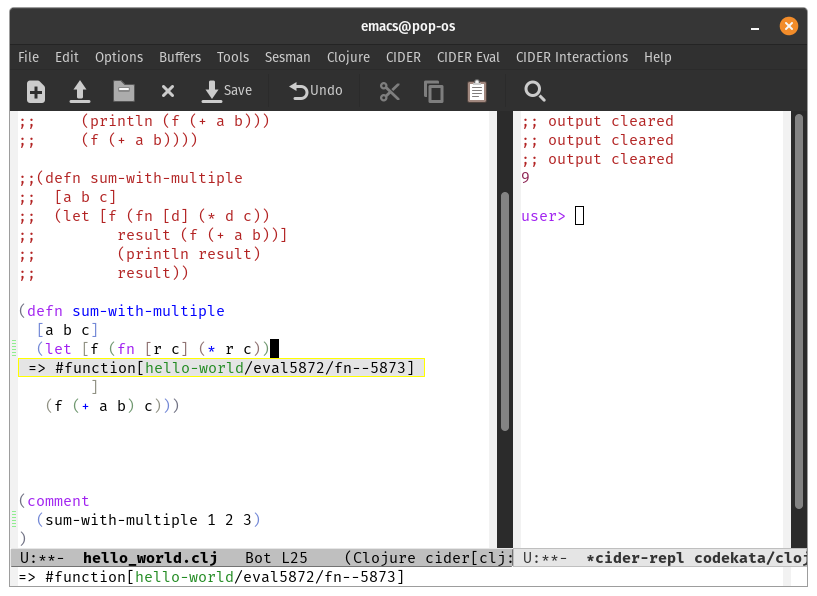Evaluated function is saved to *1. Call this function.

``````(*1 1 3)
``````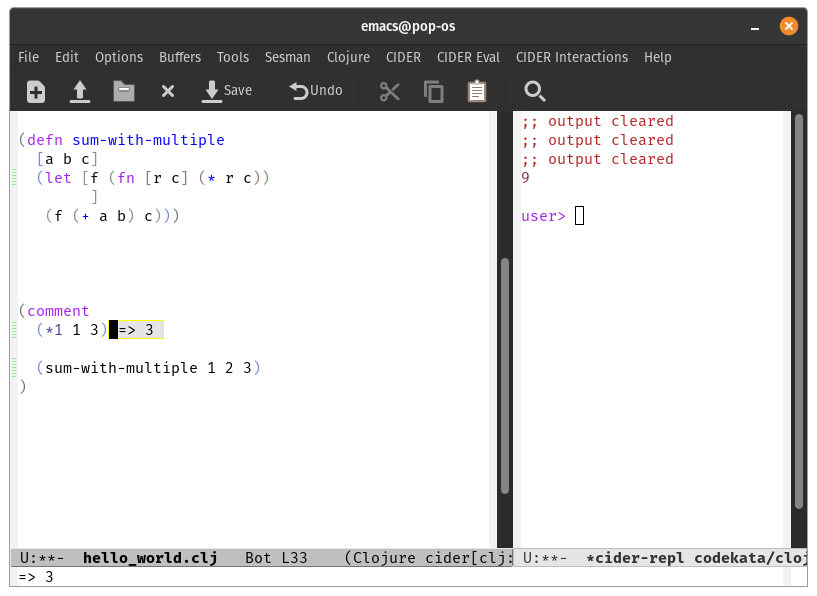Note that *1 is binding temporary.

Conclude : To use Clojure repl effectively :

• Making lambda and functions are independent
• Using thread macro like ->, ->>
• Use IDE such as VScode, intellij, emacs. (not mentioned in this article)
• When writing test code in file, write in comment clause.
• Move code from testing code in comment clause to unit test

jaeyeon-jo-kr

See other articles by jaeyeon-jo-kr

Related jobs

See all

### WorksHub

CareersCompaniesSitemapFunctional WorksBlockchain WorksJavaScript WorksAI WorksGolang WorksJava WorksPython WorksRemote Works

### Locationshello@works-hub.comGround Floor, Verse Building, 18 Brunswick Place, London, N1 6DZ108 E 16th Street, New York, NY 10003